Open In App
Related Articles

# Depth First Search or DFS for a Graph

Depth First Traversal (or DFS) for a graph is similar to Depth First Traversal of a tree. The only catch here is, that, unlike trees, graphs may contain cycles (a node may be visited twice). To avoid processing a node more than once, use a boolean visited array. A graph can have more than one DFS traversal.

Example:

Input: n = 4, e = 6
0 -> 1, 0 -> 2, 1 -> 2, 2 -> 0, 2 -> 3, 3 -> 3
Output: DFS from vertex 1 : 1 2 0 3
Explanation:
DFS Diagram: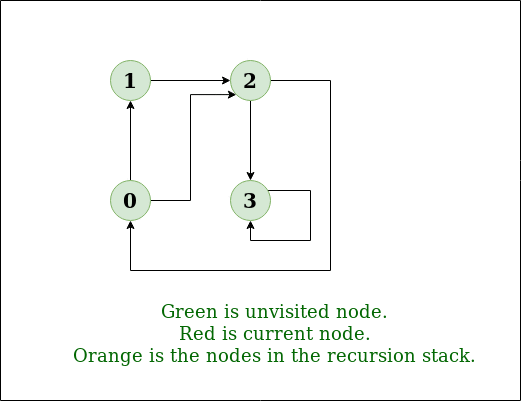Example 1

Input: n = 4, e = 6
2 -> 0, 0 -> 2, 1 -> 2, 0 -> 1, 3 -> 3, 1 -> 3
Output: DFS from vertex 2 : 2 0 1 3
Explanation:
DFS Diagram: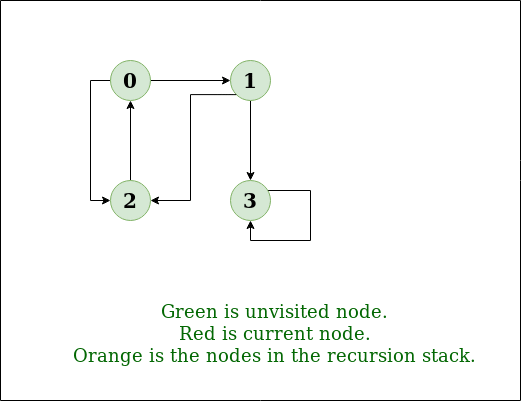Example 2

Recommended Practice

## How does DFS work?

Depth-first search is an algorithm for traversing or searching tree or graph data structures. The algorithm starts at the root node (selecting some arbitrary node as the root node in the case of a graph) and explores as far as possible along each branch before backtracking.

Let us understand the working of Depth First Search with the help of the following illustration:

Step1: Initially stack and visited arrays are empty.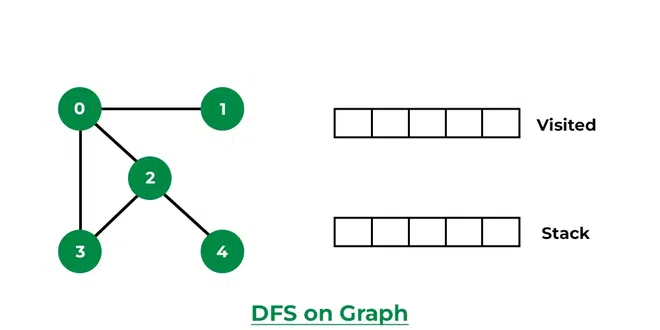Stack and visited arrays are empty initially.

Step 2: Visit 0 and put its adjacent nodes which are not visited yet into the stack.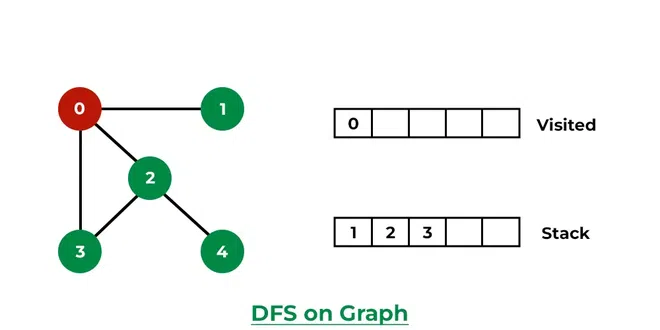Visit node 0 and put its adjacent nodes (1, 2, 3) into the stack

Step 3: Now, Node 1 at the top of the stack, so visit node 1 and pop it from the stack and put all of its adjacent nodes which are not visited in the stack.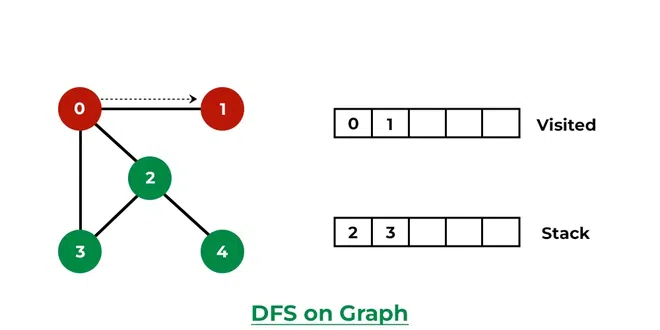Visit node 1

Step 4: Now, Node 2 at the top of the stack, so visit node 2 and pop it from the stack and put all of its adjacent nodes which are not visited (i.e, 3, 4) in the stack.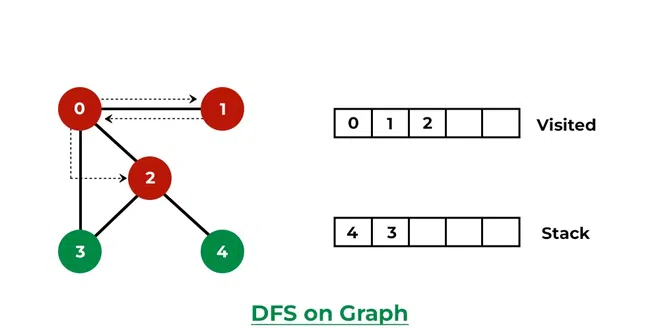Visit node 2 and put its unvisited adjacent nodes (3, 4) into the stack

Step 5: Now, Node 4 at the top of the stack, so visit node 4 and pop it from the stack and put all of its adjacent nodes which are not visited in the stack.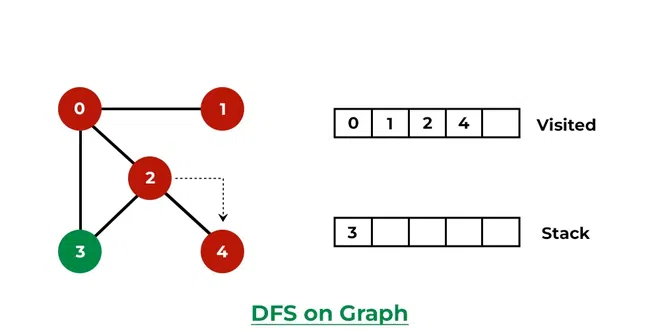Visit node 4

Step 6: Now, Node 3 at the top of the stack, so visit node 3 and pop it from the stack and put all of its adjacent nodes which are not visited in the stack.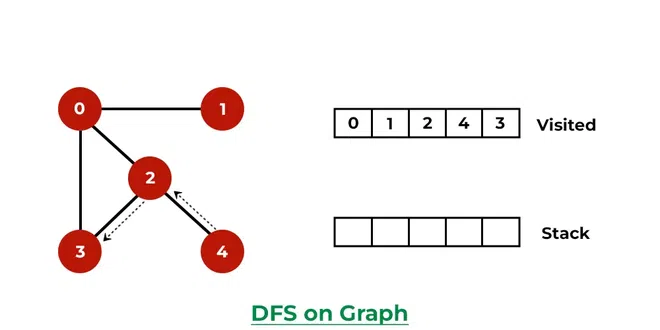Visit node 3

Now, Stack becomes empty, which means we have visited all the nodes and our DFS traversal ends.

Below is the implementation of the above approach:

## C++

 `// C++ program to print DFS traversal from``// a given vertex in a  given graph``#include ``using` `namespace` `std;` `// Graph class represents a directed graph``// using adjacency list representation``class` `Graph {``public``:``    ``map<``int``, ``bool``> visited;``    ``map<``int``, list<``int``> > adj;` `    ``// Function to add an edge to graph``    ``void` `addEdge(``int` `v, ``int` `w);` `    ``// DFS traversal of the vertices``    ``// reachable from v``    ``void` `DFS(``int` `v);``};` `void` `Graph::addEdge(``int` `v, ``int` `w)``{``    ``// Add w to v’s list.``    ``adj[v].push_back(w);``}` `void` `Graph::DFS(``int` `v)``{``    ``// Mark the current node as visited and``    ``// print it``    ``visited[v] = ``true``;``    ``cout << v << ``" "``;` `    ``// Recur for all the vertices adjacent``    ``// to this vertex``    ``list<``int``>::iterator i;``    ``for` `(i = adj[v].begin(); i != adj[v].end(); ++i)``        ``if` `(!visited[*i])``            ``DFS(*i);``}` `// Driver code``int` `main()``{``    ``// Create a graph given in the above diagram``    ``Graph g;``    ``g.addEdge(0, 1);``    ``g.addEdge(0, 2);``    ``g.addEdge(1, 2);``    ``g.addEdge(2, 0);``    ``g.addEdge(2, 3);``    ``g.addEdge(3, 3);` `    ``cout << ``"Following is Depth First Traversal"``            ``" (starting from vertex 2) \n"``;` `    ``// Function call``    ``g.DFS(2);` `    ``return` `0;``}` `// improved by Vishnudev C`

## Java

 `// Java program to print DFS traversal``// from a given graph``import` `java.io.*;``import` `java.util.*;` `// This class represents a``// directed graph using adjacency``// list representation``class` `Graph {``    ``private` `int` `V;` `    ``// Array  of lists for``    ``// Adjacency List Representation``    ``private` `LinkedList adj[];` `    ``// Constructor``    ``@SuppressWarnings``(``"unchecked"``) Graph(``int` `v)``    ``{``        ``V = v;``        ``adj = ``new` `LinkedList[v];``        ``for` `(``int` `i = ``0``; i < v; ++i)``            ``adj[i] = ``new` `LinkedList();``    ``}` `    ``// Function to add an edge into the graph``    ``void` `addEdge(``int` `v, ``int` `w)``    ``{``        ``// Add w to v's list.``        ``adj[v].add(w);``    ``}` `    ``// A function used by DFS``    ``void` `DFSUtil(``int` `v, ``boolean` `visited[])``    ``{``        ``// Mark the current node as visited and print it``        ``visited[v] = ``true``;``        ``System.out.print(v + ``" "``);` `        ``// Recur for all the vertices adjacent to this``        ``// vertex``        ``Iterator i = adj[v].listIterator();``        ``while` `(i.hasNext()) {``            ``int` `n = i.next();``            ``if` `(!visited[n])``                ``DFSUtil(n, visited);``        ``}``    ``}` `    ``// The function to do DFS traversal.``    ``// It uses recursive DFSUtil()``    ``void` `DFS(``int` `v)``    ``{``        ``// Mark all the vertices as``        ``// not visited(set as``        ``// false by default in java)``        ``boolean` `visited[] = ``new` `boolean``[V];` `        ``// Call the recursive helper``        ``// function to print DFS``        ``// traversal``        ``DFSUtil(v, visited);``    ``}` `    ``// Driver Code``    ``public` `static` `void` `main(String args[])``    ``{``        ``Graph g = ``new` `Graph(``4``);` `        ``g.addEdge(``0``, ``1``);``        ``g.addEdge(``0``, ``2``);``        ``g.addEdge(``1``, ``2``);``        ``g.addEdge(``2``, ``0``);``        ``g.addEdge(``2``, ``3``);``        ``g.addEdge(``3``, ``3``);` `        ``System.out.println(``            ``"Following is Depth First Traversal "``            ``+ ``"(starting from vertex 2)"``);` `        ``// Function call``        ``g.DFS(``2``);``    ``}``}``// This code is contributed by Aakash Hasija`

## Python3

 `# Python3 program to print DFS traversal``# from a given  graph``from` `collections ``import` `defaultdict`  `# This class represents a directed graph using``# adjacency list representation``class` `Graph:` `    ``# Constructor``    ``def` `__init__(``self``):` `        ``# Default dictionary to store graph``        ``self``.graph ``=` `defaultdict(``list``)` `    ` `    ``# Function to add an edge to graph``    ``def` `addEdge(``self``, u, v):``        ``self``.graph[u].append(v)` `    ` `    ``# A function used by DFS``    ``def` `DFSUtil(``self``, v, visited):` `        ``# Mark the current node as visited``        ``# and print it``        ``visited.add(v)``        ``print``(v, end``=``' '``)` `        ``# Recur for all the vertices``        ``# adjacent to this vertex``        ``for` `neighbour ``in` `self``.graph[v]:``            ``if` `neighbour ``not` `in` `visited:``                ``self``.DFSUtil(neighbour, visited)` `    ` `    ``# The function to do DFS traversal. It uses``    ``# recursive DFSUtil()``    ``def` `DFS(``self``, v):` `        ``# Create a set to store visited vertices``        ``visited ``=` `set``()` `        ``# Call the recursive helper function``        ``# to print DFS traversal``        ``self``.DFSUtil(v, visited)`  `# Driver's code``if` `__name__ ``=``=` `"__main__"``:``    ``g ``=` `Graph()``    ``g.addEdge(``0``, ``1``)``    ``g.addEdge(``0``, ``2``)``    ``g.addEdge(``1``, ``2``)``    ``g.addEdge(``2``, ``0``)``    ``g.addEdge(``2``, ``3``)``    ``g.addEdge(``3``, ``3``)` `    ``print``(``"Following is Depth First Traversal (starting from vertex 2)"``)``    ` `    ``# Function call``    ``g.DFS(``2``)` `# This code is contributed by Neelam Yadav`

## C#

 `// C# program to print DFS traversal``// from a given graph``using` `System;``using` `System.Collections.Generic;` `// This class represents a directed graph``// using adjacency list representation``class` `Graph {``    ``private` `int` `V;` `    ``// Array of lists for``    ``// Adjacency List Representation``    ``private` `List<``int``>[] adj;` `    ``// Constructor``    ``Graph(``int` `v)``    ``{``        ``V = v;``        ``adj = ``new` `List<``int``>[ v ];``        ``for` `(``int` `i = 0; i < v; ++i)``            ``adj[i] = ``new` `List<``int``>();``    ``}` `    ``// Function to Add an edge into the graph``    ``void` `AddEdge(``int` `v, ``int` `w)``    ``{``        ``// Add w to v's list.``        ``adj[v].Add(w);``    ``}` `    ``// A function used by DFS``    ``void` `DFSUtil(``int` `v, ``bool``[] visited)``    ``{``        ``// Mark the current node as visited``        ``// and print it``        ``visited[v] = ``true``;``        ``Console.Write(v + ``" "``);` `        ``// Recur for all the vertices``        ``// adjacent to this vertex``        ``List<``int``> vList = adj[v];``        ``foreach``(``var` `n ``in` `vList)``        ``{``            ``if` `(!visited[n])``                ``DFSUtil(n, visited);``        ``}``    ``}` `    ``// The function to do DFS traversal.``    ``// It uses recursive DFSUtil()``    ``void` `DFS(``int` `v)``    ``{``        ``// Mark all the vertices as not visited``        ``// (set as false by default in c#)``        ``bool``[] visited = ``new` `bool``[V];` `        ``// Call the recursive helper function``        ``// to print DFS traversal``        ``DFSUtil(v, visited);``    ``}` `    ``// Driver Code``    ``public` `static` `void` `Main(String[] args)``    ``{``        ``Graph g = ``new` `Graph(4);` `        ``g.AddEdge(0, 1);``        ``g.AddEdge(0, 2);``        ``g.AddEdge(1, 2);``        ``g.AddEdge(2, 0);``        ``g.AddEdge(2, 3);``        ``g.AddEdge(3, 3);` `        ``Console.WriteLine(``            ``"Following is Depth First Traversal "``            ``+ ``"(starting from vertex 2)"``);` `        ``// Function call``        ``g.DFS(2);``        ``Console.ReadKey();``    ``}``}` `// This code is contributed by techno2mahi`

## Javascript

 `// Javascript program to print DFS``// traversal from a given``// graph` `// This class represents a``// directed graph using adjacency``// list representation``class Graph``{``    ` `    ``// Constructor``    ``constructor(v)``    ``{``        ``this``.V = v;``        ``this``.adj = ``new` `Array(v);``        ``for``(let i = 0; i < v; i++)``            ``this``.adj[i] = [];``    ``}``    ` `    ``// Function to add an edge into the graph``    ``addEdge(v, w)``    ``{``        ` `        ``// Add w to v's list.``        ``this``.adj[v].push(w);``    ``}``    ` `    ``// A function used by DFS``    ``DFSUtil(v, visited)``    ``{``        ` `        ``// Mark the current node as visited and print it``        ``visited[v] = ``true``;``        ``console.log(v + ``" "``);`` ` `        ``// Recur for all the vertices adjacent to this``        ``// vertex``        ``for``(let i of ``this``.adj[v].values())``        ``{``            ``let n = i``            ``if` `(!visited[n])``                ``this``.DFSUtil(n, visited);``        ``}``    ``}``    ` `    ``// The function to do DFS traversal.``    ``// It uses recursive``    ``// DFSUtil()``    ``DFS(v)``    ``{``        ` `        ``// Mark all the vertices as``        ``// not visited(set as``        ``// false by default in java)``        ``let visited = ``new` `Array(``this``.V);``        ``for``(let i = 0; i < ``this``.V; i++)``            ``visited[i] = ``false``;`` ` `        ``// Call the recursive helper``        ``// function to print DFS``        ``// traversal``        ``this``.DFSUtil(v, visited);``    ``}``}` `// Driver Code``g = ``new` `Graph(4);`` ` `g.addEdge(0, 1);``g.addEdge(0, 2);``g.addEdge(1, 2);``g.addEdge(2, 0);``g.addEdge(2, 3);``g.addEdge(3, 3);` `console.log(``"Following is Depth First Traversal "` `+``               ``"(starting from vertex 2)"``);` `g.DFS(2);` `// This code is contributed by avanitrachhadiya2155`

Output

```Following is Depth First Traversal (starting from vertex 2)
2 0 1 3 ```

Time complexity: O(V + E), where V is the number of vertices and E is the number of edges in the graph.
Auxiliary Space: O(V + E), since an extra visited array of size V is required, And stack size for iterative call to DFS function.